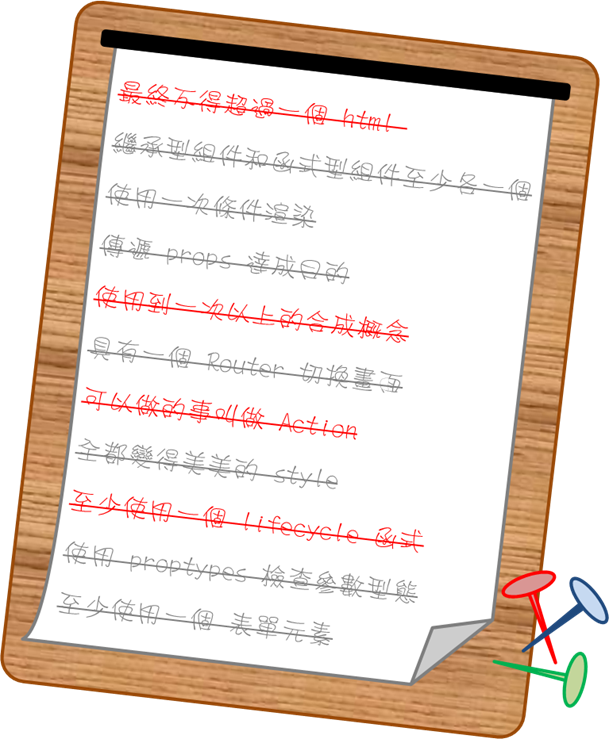#2018 iT 邦幫忙鐵人賽DAY 30
0
Modern Web

## 【Day30】 30天 一步一腳印

``````// reducer.js
function insertResult(array, result){
var i = array.length;
return [
...array.slice(0,i),
result
];
}
``````

Store 持有的是預設資料而不是隨機資料

``````function getNewNumber(){
var abGame = [0,1,2,3,4,5,6,7,8,9];
var i, j, swap, result;
var k = Math.floor(Math.random()*6);

for(i=0; i<9; i++){
j = Math.floor(Math.random()*9);
swap = abGame[i];
abGame[i] = abGame[j];
abGame[j] = swap;
}
result = abGame.slice(k, k+4);
if( result == 0 ){
j = Math.floor(Math.random()*2+1);
swap = result;
result = result[j];
result[j] = swap;
}
return result;
}
``````

``````class AB extends React.Component{
constructor(){
super();
}

componentDidMount(){
this.props.playAgainAABB();
}

render(){
var textStyle = {
}

var newGameStyle = {
display:'inline-block',
marginLeft:'40px'
}

return(
<div className="AB">
<div className="left">
<fieldset>
<legend>猜題紀錄</legend>
{this.props.History.map(
(History, i) => <ABList key={i} data={History} />
)}
</fieldset>
</div>
<div className="right">
<h3>規則：</h3>
<p style={textStyle}>數字對位置對為A</p>
<p style={textStyle}>數字對位置錯為B</p>
<p style={textStyle}>數字不會重複出現</p>
<p style={textStyle}>數字0不會出現在第一位</p>
<input type="text" placeholder="請輸入4位不重複數字"
onChange={(event) => this.props.saveInput(event)} maxLength="4"/>
<input type="button" value="確認" onClick={this.props.guessNum}/>
<input style={newGameStyle} type="button" value="新遊戲" onClick={this.props.playAgainAABB} />
</div>
</div>
);
}
}
``````

`componentDidMount`中呼叫一次初始化的 Action

###### >>> 隊友任意門 <<<

Day30 end
by 瑞Ray (((o(ﾟ▽ﾟ)o)))